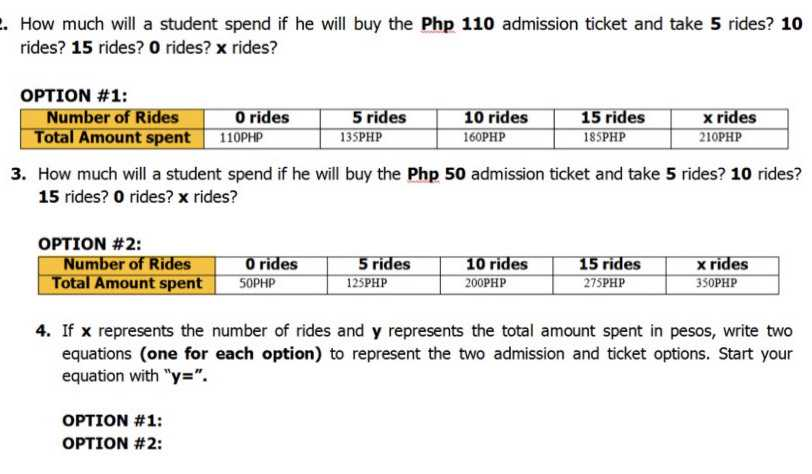### ¿Todavía tienes preguntas de matemáticas?

Pregunte a nuestros tutores expertos
Algebra
Pregunta2. How much will a student spend if he will buy the Php $$110$$ admission ticket and take $$5$$ rides? $$10$$ rides? $$15$$ rides? $$0$$ rides? $$x$$ rides?

3. How much will a student spend if he will buy the Php $$5 0$$ admission ticket and take $$5$$ rides? $$10$$ rides? $$1 5$$ rides? $$0$$ rides? $$x$$ rides?

4. If $$x$$ represents the number of rides and $$y$$ represents the total amount spent in pesos, write two equations (one for each option) to represent the two admission and ticket options. Start your equation with " $$y = "$$ .

option #1: $$y= 5x+ 110$$
option #2: $$y= 15x+ 50$$